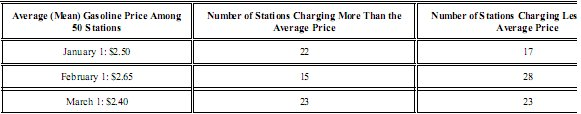#The table above shows the average price of gasoline among 50 gasoline-service stations on three different days, along with the number of stations charging more and less than the average on each day.Based on the information in the table, which of the following statements about the price of gasoline among all 50 stations is NOT true?

(1) On March 1, the average prime was equal to the median price.

Explanation

The correct answer is (1). The statement in choice (1) is not necessarily true. On March 1, the number of stations charging more than the average price was the same as the number of stations charging less than the average price; the median pricemis the average of the two middle prices, which may or may not be the same as the average of all 50 prices. The statement in choice (2) must be true. On February 1, more stations charged less than the average price than greater than the average price; in other words, the median price was less than the average price. The statement in choice (3) must be true. The mecan price on March 1 was the same as the average price: $$\2.40$$. The median price on January 1 was greater than the average price of $$\2.50$$. The statement in choice (4) must be true. The average price on February 1 was $$\2.65$$, more than an average price of $$\2.50$$ on January 1. The statement in choice (5) must be true. On January 1, 6 stations charged the average price for that day, whereas on February 1, the number was 8.